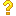•闪存
•博客
• 发言小组
• 投递新闻
• 提问博问
• 添加收藏
• 发布招聘
•文库

0

##40matlab练习题求解

jzi=input(''); if jzi>=1 && jzi<=20 for f1=1:1:jzi a(f1,:)=input(''); %操作f1行所有数据 end for f2=1:1:jzi
0

1

6